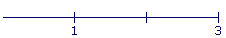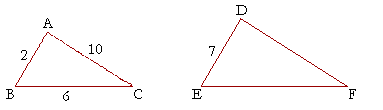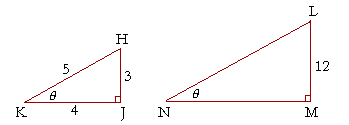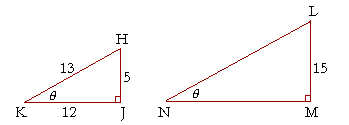1

# RATIO AND PROPORTION

The natural numbers:  Cardinal and ordinal

Parts of natural numbers

Parts, plural

The ratio of natural numbers

The names of the fractions

Proportions

The theorem of the alternate proportion

The theorem of the same multiple

Similar figures

TRIGONOMETRY historically is the study of triangles. The name literally means measurement of triangles. It begins with the study of right triangles, which are triangles that have a right angle, and with the ratios-- the relationships -- of their sides. The meaning of a ratio depends on what we mean by the parts of a number. And we name parts with ordinal numbers: the third part, the fourth part, the fifth, and so on. So with those we shall begin.

Natural numbers:  cardinal and ordinal

The natural numbers are the counting numbers.  They have two forms, cardinal and ordinal. The cardinal forms are

One, two, three, four, and so on.

They answer the question How much? or  How many?.  The ordinal forms are

First, second, third, fourth, and so on.

They answer the question Which one?.

Parts of natural numbers

If a larger number is a multiple of a smaller number, we say that the smaller number is a part of the larger.

Here are the first few multiples of 5:

5, 10, 15, 20, 25, 30, 35.

5 is the first multiple of 5.  10 is the second multiple;  15, the third; and so on.

5 is a part of each one of those, except itself.

Now, since 15, for example, is the third multiple of 5, we say that 5 is the third part of 15.  We use that same ordinal number to name the part.

5 is the fourth part of 20.  It is the fifth part of 25; the sixth part of 30.  And so on.

5 is which part of 10? We do not say the second part. We say half.  5 is half of 10.

So with the exception of half, we name the parts with ordinal numbers.  Each ordinal number names which part.

Again, 5 is not a part of itself.  There is no such thing as the first part.  (See Skill in Arithmetic, Lesson 15.)

It is important to understand that we are not speaking here of proper fractions: numbers that are less than 1 and that we need for measuring. We are explaining how the ordinal numbers—third, fourth, fifth, and so on—name the parts of the cardinal numbers.  We will come to those fractional symbols shortly.

Problem 1.   7 is which part of 28?    The fourth.

Problem 2.   Which part of 45 is 9?   The fifth.

Problem 3.   6 is which part of 12?    Half.

Problem 4.   What number is the eighth part of 24?    3

Problem 5.   3 is the tenth part of what number?    30

Parts, pluralThe figure shows that we have divided 15 into thirds, that is, into three equal parts, and that each 5 is a third part of 15.

5,  10,  15.

Therefore, 10 -- which is two 5's -- is two third parts of 15. One third. Two thirds.  5,  10.

Now, 10 is not a part of 15, because 15 is not a multiple of 10.  We say that it is parts of 15, plural:  Two third parts, or simply two thirds.  Those words are to be taken literally.

Similarly, if we divide 15 into fifths, that is, into five equal parts:-- then 3 is the fifth part of 15.

6 is two fifth parts of 15.  9 is three fifths of 15.  12 is four fifths.  And 15 is all five of its fifth parts.

We can now say what we mean by

The ratio of natural numbers

DEFINITION.  The ratio of two natural numbers is their relationship with respect to relative size, which we can always name. Specifically, we can say that one number is a multiple of the other (so many times it), a part of it, or parts of it.

Example 1.  Multiple   What ratio has 15 to 5?

Answer.  15 is three times 5.

That is the ratio -- the relationship -- of 15 to 5.

We do not answer "3 to 1," because we want to name the ratio of 15 to 5 explicitly.  It is true that 15 has the same ratio to 5 that 3 has to 1. 3 is three times 1, just as 15 is three times 5.

The two numbers in a ratio are called the terms; the first term and the second.

Notice that we answer with a complete sentence beginning with the first term and ending with the second:  "15 is three times 5."  For, a ratio is a relationship.

Example 2.  Part   What ratio has 5 to 15?

Answer.  5 is the third part of 15.

That is the inverse ratio of 15 to 5.  The terms are exchanged.

Example 3.  Parts  What ratio has 10 to 15?

Answer.  10 is two thirds of 15.Those are the three kinds of ratio: One number is a multiple of the other -- so many times it -- a part of it, or parts of it.

Problem 6.   What ratio have the following?  Answer with a complete sentence beginning with the first term.

a)   2 to 10?   2 is the fifth part of 10.

b)   10 to 2?   10 is five times 2.

A larger number will always be so many times a smaller.

c)   7 to 1?   7 is seven times 1.

d)   1 to 7?   1 is the seventh part of 7.

e)   25 to 100?   25 is the fourth part of 100.

f)   75 to 100?   75 is three fourths of 100.

g)   12 to 6?   12 is two times 6, or twice as much as 6, or double 6.

h)   6 to 12?   6 is half of 12.

The names of the fractions

In English, the proper fractions have the same names as the ratio of the numerator to the denominator.  The number we write as 1 over 3 ---- is called "one-third" because of the ratio of 1 to 3.  1 is one third of 3.And the fractionhas that same ratio to 1.For that reason, fractions are called rational numbers.

Rational numbers have the same ratio to 1 as two natural numbers.

Fractional symbols may therefore be regarded as ratio symbols, in that they signify the ratio of the numerator to the denominator.

 12 = 24 = 5 10 = 9 18 , etc.

1 is to 2  as  2 is to 4  as  5 is to 10, etc.

In these equivalent fractions, each numerator is half of its denominator.

Example 4.   What ratio has 3 to 4?

Answer.   We can express the ratio of any smaller number to a larger simply by letting each number say its name.  3 is three fourths of 4. 3 says its cardinal name "three." 4 says its ordinal name "fourths."

On the practical side, since the student has very likely learned the names of the fractions first, then the ratio of 3 to 4 is expressed by the name of the fraction.  3 is three fourths of 4.

Proportions

A proportion is a statement that two ratios are the same.

5 is to 15  as  8 is to 24.

5 is the third part of 15, just as 8 is the third part of 24.

We will now introduce this symbol  5 : 15  to signify the ratio of 5 to 15.  A proportion will then appear as follows:

5 : 15 = 8 : 24.

"5 is to 15  as  8 is to 24."

Or, we can represent a proportion with fractional symbols --

 5 15 = 8 24

-- and read it the same way:

"5 is to 15  as  8 is to 24."

The four numbers in a proportion are called the terms. The 1st, the 2nd, the 3rd, the 4th.

Example 5.   12 : 2 = 42 : 7.  ("12 is to 2  as  42 is to 7.")

Why is this a proportion?

Answer.  Because 12 is six times 2, just as 42 is six times 7.

If the student will say the ratio of the 1st term to the 2nd, the answer will be clear.

Problem 7.   Why is each of these a proportion?

a)  10 : 2 = 5 : 1.   10 is five times 2, just as 5 is five times 1.

b)  3 : 9 = 4 : 12.   3 is the third part of 9. 4 is the third part of 12.

c)  10 : 15 = 2 : 3.   10 is two thirds of 15. 2 is two thirds of 3.Problem 8.   Complete each proportion.

 a)   2 : 8 = 9 : 36 b)   14 : 2 = 35 : 5 c)   3 : 4 = 75 : 100 d)   5 : 15 = 8 : 24 e)   72 : 9 = 16 : 2 f)   2 : 3 = 40 : 60

The theorem of the alternate proportion

Here is a proportion:

1st : 2nd = 3rd : 4th

We say that the 1st and the 3rd are corresponding terms, as are the 2nd and the 4th.

The following is the theorem of the alternate proportion:

 If four numbers are proportional, then the corresponding terms are also proportional. That is, as the first term is to the third, so the second will be to the fourth. If a : b = m : n, then, alternately, a : m = b : n.

Since

1 : 3 = 5 : 15,

then alternately,

1 : 5 = 3 : 15.

Example 6.   Complete this proportion:

4 : 5 = 12 : ?

4 is four fifths ("") of 5, but it is not obvious what number 12 is four fifths of.

Alternately, however, 4 is a third of 12 -- or we could say that 4 has been multiplied by 3.  Therefore 5 also must be multiplied by 3,

4 : 5 = 12 : 15

That is,

4 : 5 = 3 × 4 : 3 × 5As one 4 is to one 5, so any number of 4's will be to an equal number of 5's.  Three 4's are four fifths of three 5's.

This is the theorem of the same multiple.

 If we multiply two numbers by the same number, then the products will have the same ratio as the numbers we multiplied.

Formally,

a : b = ma : mb.

In arithmetic and algebra, this appears as the principle of equivalent fractions:

 ab = mamb

Example 7.   Complete this proportion, 5 : 8 = 35 : ?

Answer.  Look at it alternately.  35 is seven times 5.  Therefore the missing term will be seven times 8.  56.

Example 8.   Solve this proportion -- 8 : 12 = 2 : ?

Answer.  To produce 2,  8 has been divided by 4.  Therefore 12 also must be divided by 4.

8 : 12 = 2 : 3

The theorem of the same multiple may be inverted to mean that we may divide both terms by the same number.

An all too common method these days is to make this an algebra problem.

 8 12 = 2x

The student is taught to cross-multiply and solve for x. That is a method for people who do not understand ratio and proportion. It is taught in order not to teach ratio and proportion verbally. It stems from a 19th century resistance to language in mathematics.

We shall often make use of this basic property of the square root radical:(See Lesson 26 of Algebra, Radicals.)Problem 9.   Complete each proportion.

 a)   3 : 5 = 18 : 30 b)   7 : 9 = 21 : 27 c)   11 : 2 = 55 : 10 d)   14 : 35 = 2 : 5 e)   18 : 21 = 6 : 7 f)   2 : 7 = 10 : 35 g)   √3 : 2 = 3 : 2√3 h)   2 : √2 = 2√5 : √10 i)   √2 : 1 = 1 : ½√2

Similar figures

Trigonometry depends on the meaning of similar figures.

Similar figures are equiangular, and the sides that make the equal angles are proportional.To say then that figures ABCDE,  PQRST are similar, is to say that the angle at A is equal to the angle at P,  the angle at B is equal to the angle at Q, etc.;  and, proportionally, as AB is to BC, so PQ is to QR.

And so on, for each pair of equal angles and the sides that make them.

For triangles to be similar, however, it is sufficient that they be equiangular. (Theorem 15 of  "Some Theorems of Plane Geometry.") From that it follows:

Right triangles will be similar if an acute angle of one
is equal to an acute angle of the other.In the right triangles ABC, DEF, if the acute angle at B is equal to the acute angle at E, then those triangles will be similar.  Therefore the sides that make the equal angles will be proportional.  For example,

BC : CA = EF : FD.

And alternately, the corresponding sides are proportional:

BC : EF = CA : FD.

Thus right triangles will be similar if an acute angle of one is equal to an acute angle of the other. We will see that that is the basis of triangle trigonometry.

Example 10.Triangles ABC, DEF are similar, with the angle at A equal to the angle at D, the angle at B equal to the angle at E, and the angle at C equal to the angle at F.

AB = 2 cm, BC = 6 cm, CA = 10 cm, and DE = 7 cm. How long ar EF and FD?

Answer.   The sides that make the equal angles are proportional.

Since AB is is third of BC, then DE is also a third of EF. Therefore EF = 21 cm.

Next, since AC is five times AB, then DF is also five times DE.  DF = 35 cm.

Example 11.  The right triangles HJK, LMN are similar. HJ = 3 cm,JK = 4 cm, and KH = 5 cm.  If LM = 12 cm, then how long are MN and NL?

3 : 4 = 12 : MN.

To produce 12,  3 has been multiplied by 4. Therefore 4 will also be multiplied by 4. MN is 16 Cm.

Similarly, 5 will be multiplied by 4. NL is 20 cm.

In other words, since the side HJ has been multiplied by 4, then every side will be multiplied by 4.

3 : 4 : 5 = 12 : 16 : 20.

Problem 10.  Triangles ABC, DEF are similar, as in Example 10.AB = 7 cm, BC = 21 cm, CA = 14 cm, and DE = 8 cm. How long are EF and FD?

AB is the third part of BC. Therefore, DE is also the third part of EF.  EF = 24 cm.

BA is half of AC. Therefore, ED is also half of DF.  DF = 16 cm.

Problem 11.  The right triangles HJK, LMN are similar. HJ = 5 cm,JK = 12 cm, and KH = 13 cm.  If LM = 15 cm, then how long are MN and NL?

The side HJ has been multiplied by 3. Therefore every side will be multiplied by 3.

MN = 36 cm. NL = 39 cm.

*

Our definition of a proportion has been in terms of the ratio of natural numbers. The sides of a triangle, however, are not natural numbers. They are lengths, which are magnitudes -- things that have size -- they are not numbers. And magnitudes do not necessarily have the same ratio to one another as natural numbers. They can be "incommensurable." Hence a new theory of ratio and proportion is required which will be applicable to magnitudes. The interested student is referred to Topic 11 of The Evolution of the Real Numbers, in particular the section "The new theory of proportions."

Next Topic:  The Circle

Please make a donation to keep TheMathPage online.
Even \$1 will help.